# O ADVANCED MATERIAL Identifying the major species in weak acid or weak base equilibria The preparations...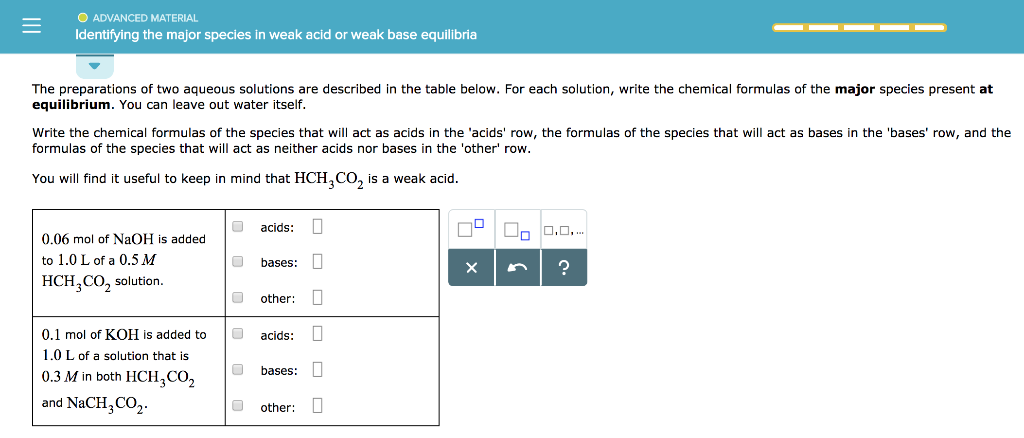O ADVANCED MATERIAL Identifying the major species in weak acid or weak base equilibria The preparations of two aqueous solutions are described in the table below. For each solution, write the chemical formulas of the major species present at equilibrium. You can leave out water itself. Write the chemical formulas of the species that will act as acids in the 'acids' row, the formulas of the species that will act as bases in the 'bases' row, and the formulas of the species that will act as neither acids nor bases in the 'other' row. You will find it useful to keep in mind that HCH,CO, is a weak acid. acids: 0 ....... 0.06 mol of NaOH is added to 1.0 L of a 0.5 M HCH,CO, solution. bases: 0 other: 0 acids: 0 0.1 mol of KOH is added to 1.0 L of a solution that is 0.3 M in both HCH,CO2 and NaCH, CO2 bases: 0 other: 0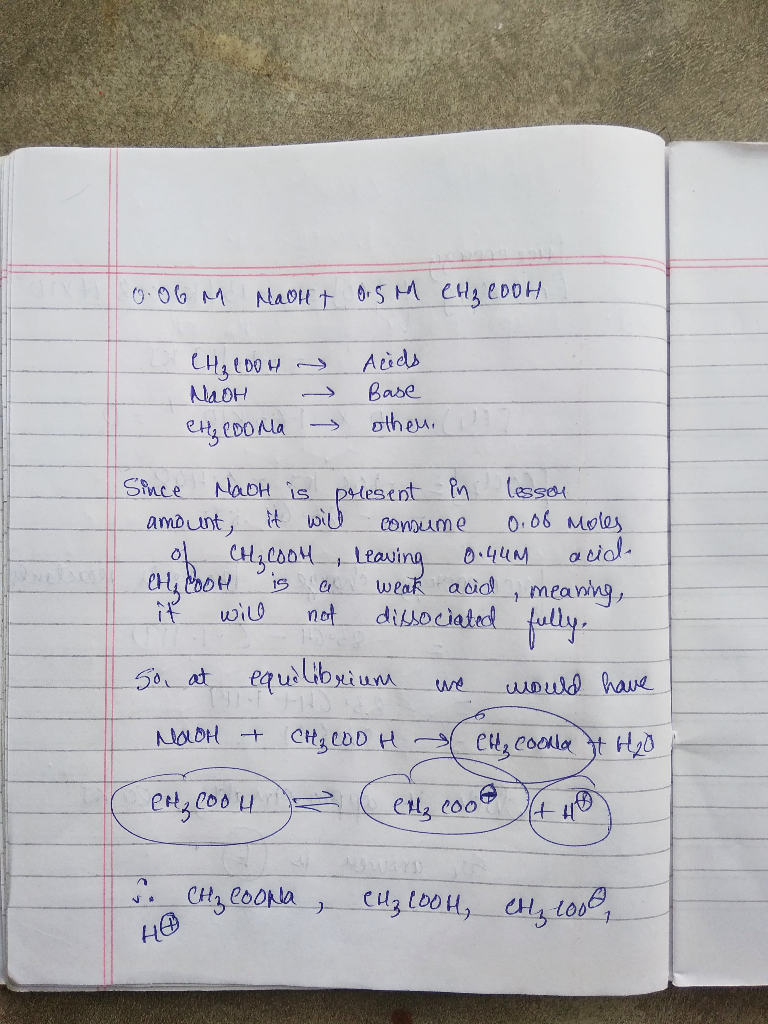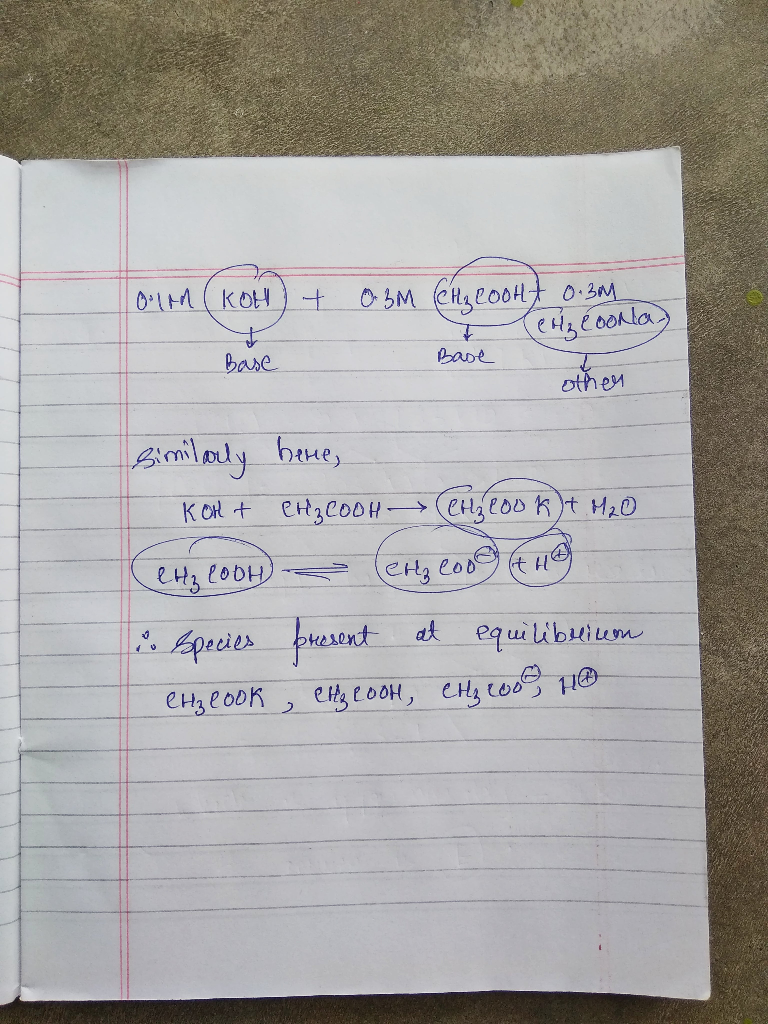#### Earn Coin

Coins can be redeemed for fabulous gifts.

Similar Homework Help Questions
• ### ACIDS AND BASES Identifying the major specles In weak acid or weak base equilib... The preparations...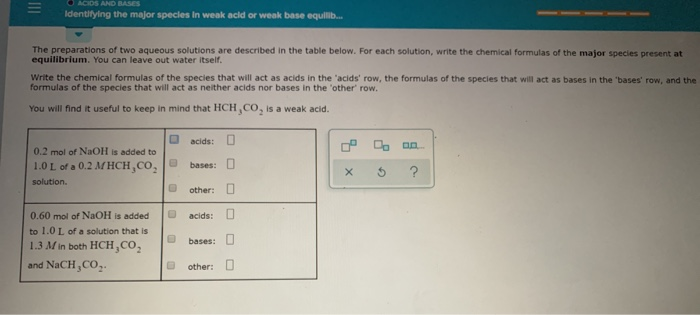ACIDS AND BASES Identifying the major specles In weak acid or weak base equilib... The preparations of two aqueous solutions are described in the table below. For each solution, write the chemical formulas of the major species present at equilibrium. You can leave out water itself. Write the chemical formulas of the species that will act as acids in the 'acids' row, the formulas of the species that will act as bases in the 'bases formulas of the species that...

• ### Identifying the major species in week acid or weak base equilibria The preparations of two aqueous...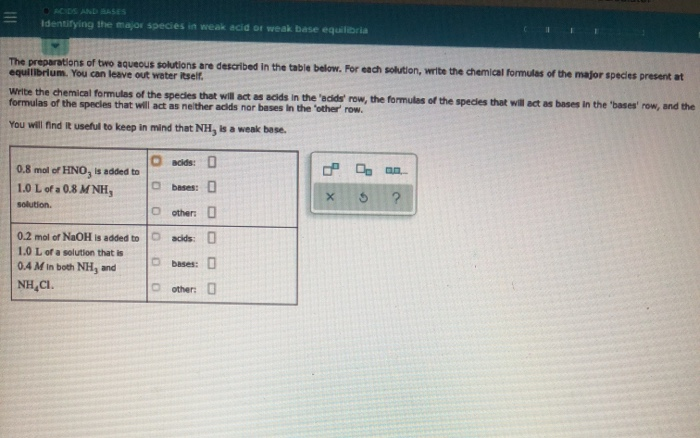Identifying the major species in week acid or weak base equilibria The preparations of two aqueous solutions are described in the table below. For each solution, write the chemical formules of the major species present at equilibrium. You can leave oue water itselt. Write the chemical formulas of the species that will act as acids in the 'acids' row, the formules of the species that will act as bases in the bases' row, and the formulas of the species that...

• ### - ACIDS AND BASES Identifying the major species in weak acid or weak base The preparations...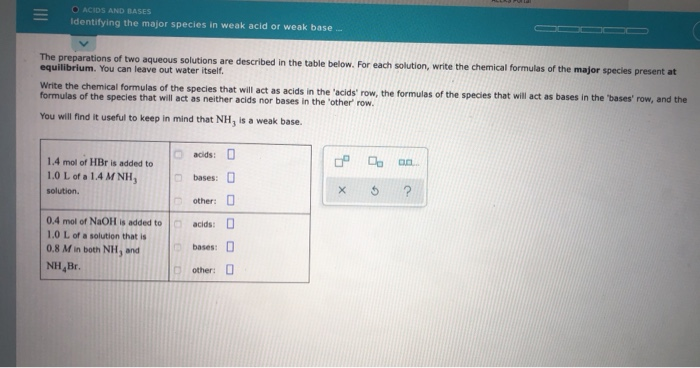- ACIDS AND BASES Identifying the major species in weak acid or weak base The preparations of two aqueous solutions are described in the table below. For each solution, write the chemical formulas of the major species present at equilibrium. You can leave out water itself Write the chemical formulas of the species that will act as acids in the 'acids' row, the formulas of the species that will act as bases in the bases' row, and the formulas of...

• ### Identifying the major species in weak acid or weak base equilib... The preparations of two aqueous...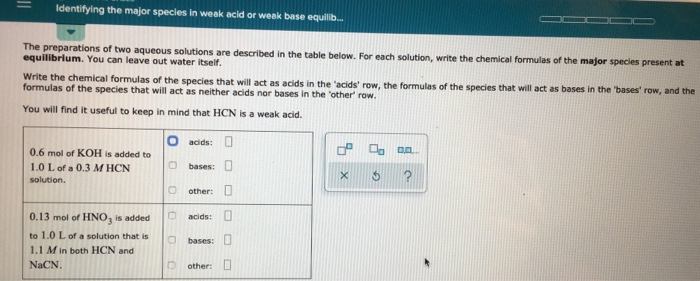Identifying the major species in weak acid or weak base equilib... The preparations of two aqueous solutions are described in the table below. For each solution, write the chemical formulas of the major species present at equilibrium. You can leave out water itself. Write the chemical formulas of the species that will act as acids in the 'acids' row, the formulas of the species that will act as bases in the bases row, and the formulas of the species that...

• ### The preparations of two aqueous solutions are described in the table below. For each solution, write...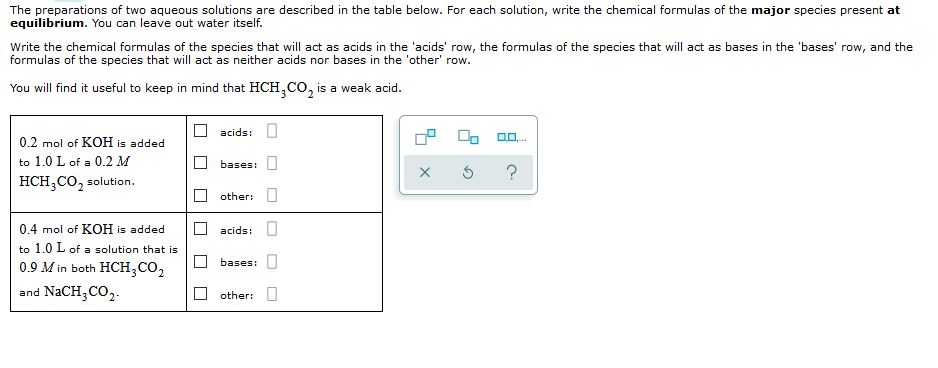The preparations of two aqueous solutions are described in the table below. For each solution, write the chemical formulas of the major species present at equilibrium. You can leave out water itself. Write the chemical formulas of the species that will act as acids in the 'acids' row, the formulas of the species that will act as bases in the 'bases' row, and the formulas of the species that will act as neither acids nor bases in the 'other' row....

• ### The preparations of two aqueous solutions are described in the table below. For each solution, wr...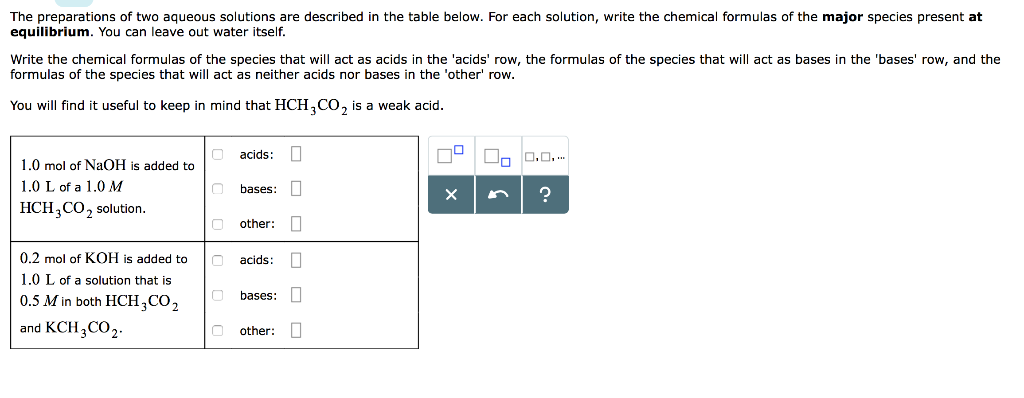The preparations of two aqueous solutions are described in the table below. For each solution, write the chemical formulas of the major species present at equilibrium. You can leave out water itself. Write the chemical formulas of the species that will act as acids in the 'acids' row, the formulas of the species that will act as bases in the 'bases' row, and the formulas of the species that will act as neither acids nor bases in the 'other row....

• ### The preparations of two aqueous solutions are described in the table below. For each solution, write the chemical f...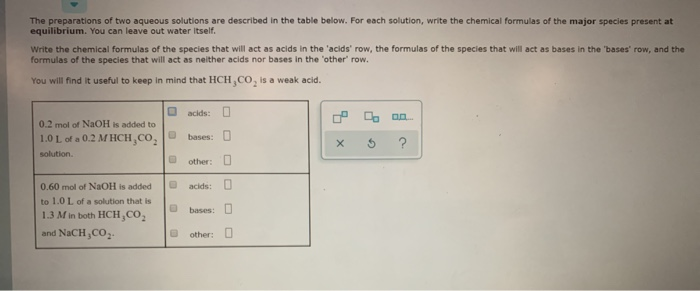The preparations of two aqueous solutions are described in the table below. For each solution, write the chemical formulas of the major species present at equilibrium. You can leave out water itself. Write the chemical formulas of the species that will act as acids in the 'acids' row, the formulas of the species that will act as bases in the 'bases' row, and the formulas of the species that will act as neither acids nor bases in the 'other' row....

• ### The preparations of two aqueous solutions are described in the table below. For each solution, write...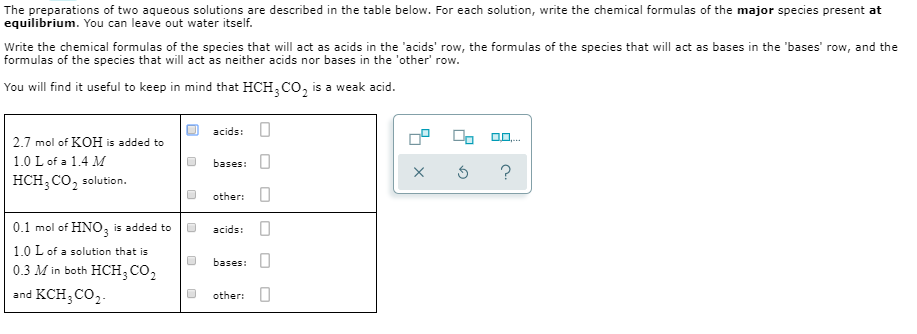The preparations of two aqueous solutions are described in the table below. For each solution, write the chemical formulas of the major species present at equilibrium. You can leave out water itself. Write the chemical formulas of the species that will act as acids in the 'acids' row, the formulas of the species that will act as bases in the 'bases' row, and the formulas of the species that will act as neither acids nor bases in the 'other' row....

• ### The preparations of two aqueous solutions are described in the table below. For each solution, write...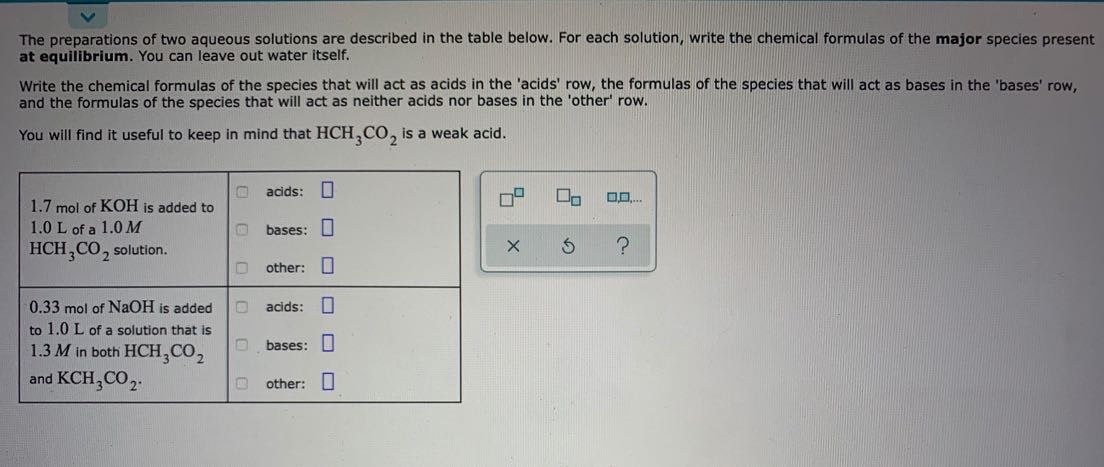The preparations of two aqueous solutions are described in the table below. For each solution, write the chemical formulas of the major species present at equilibrium. You can leave out water itself. Write the chemical formulas of the species that will act as acids in the 'acids' row, the formulas of the species that will act as bases in the 'bases' row, and the formulas of the species that will act as neither acids nor bases in the 'other' row....

• ### The preparations of two aqueous solutions are described in the table below. For each solution, write...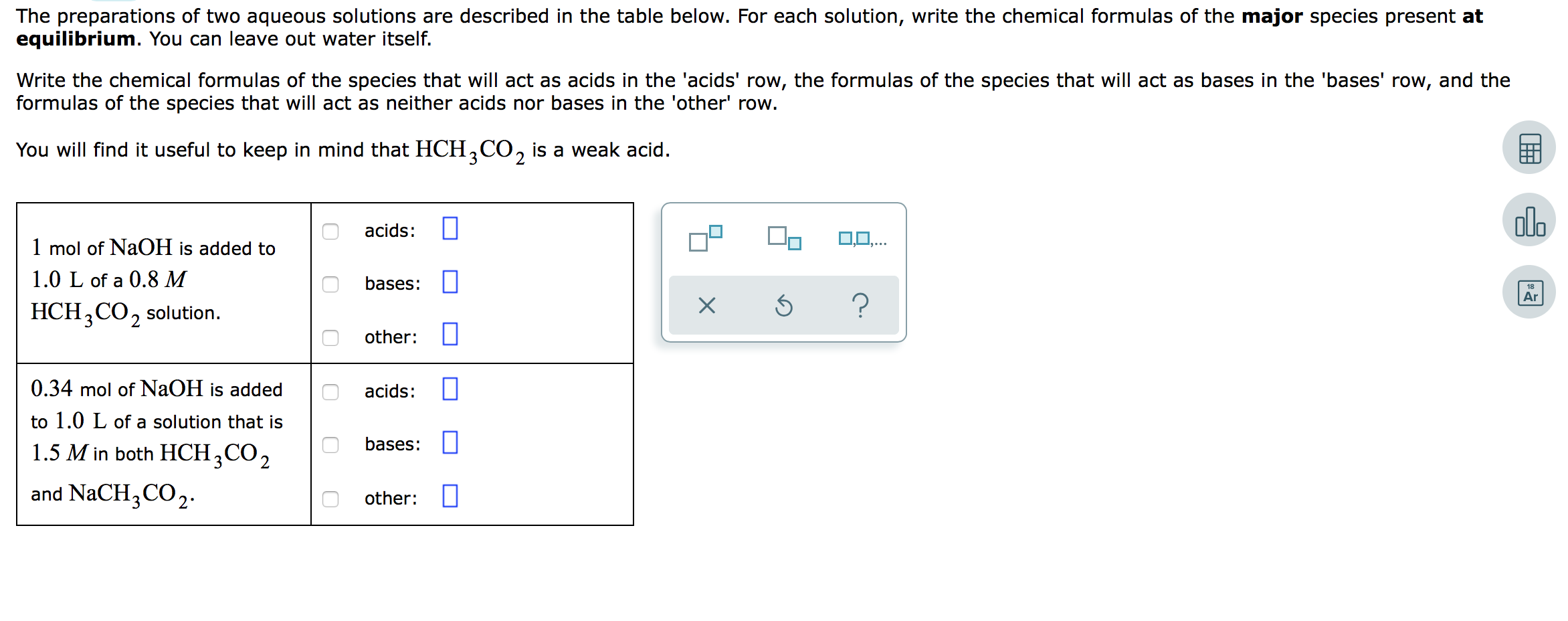The preparations of two aqueous solutions are described in the table below. For each solution, write the chemical formulas of the major species present at equilibrium. You can leave out water itself. Write the chemical formulas of the species that will act as acids in the 'acids' row, the formulas of the species that will act as bases in the 'bases' row, and the formulas of the species that will act as neither acids nor bases in the 'other' row....